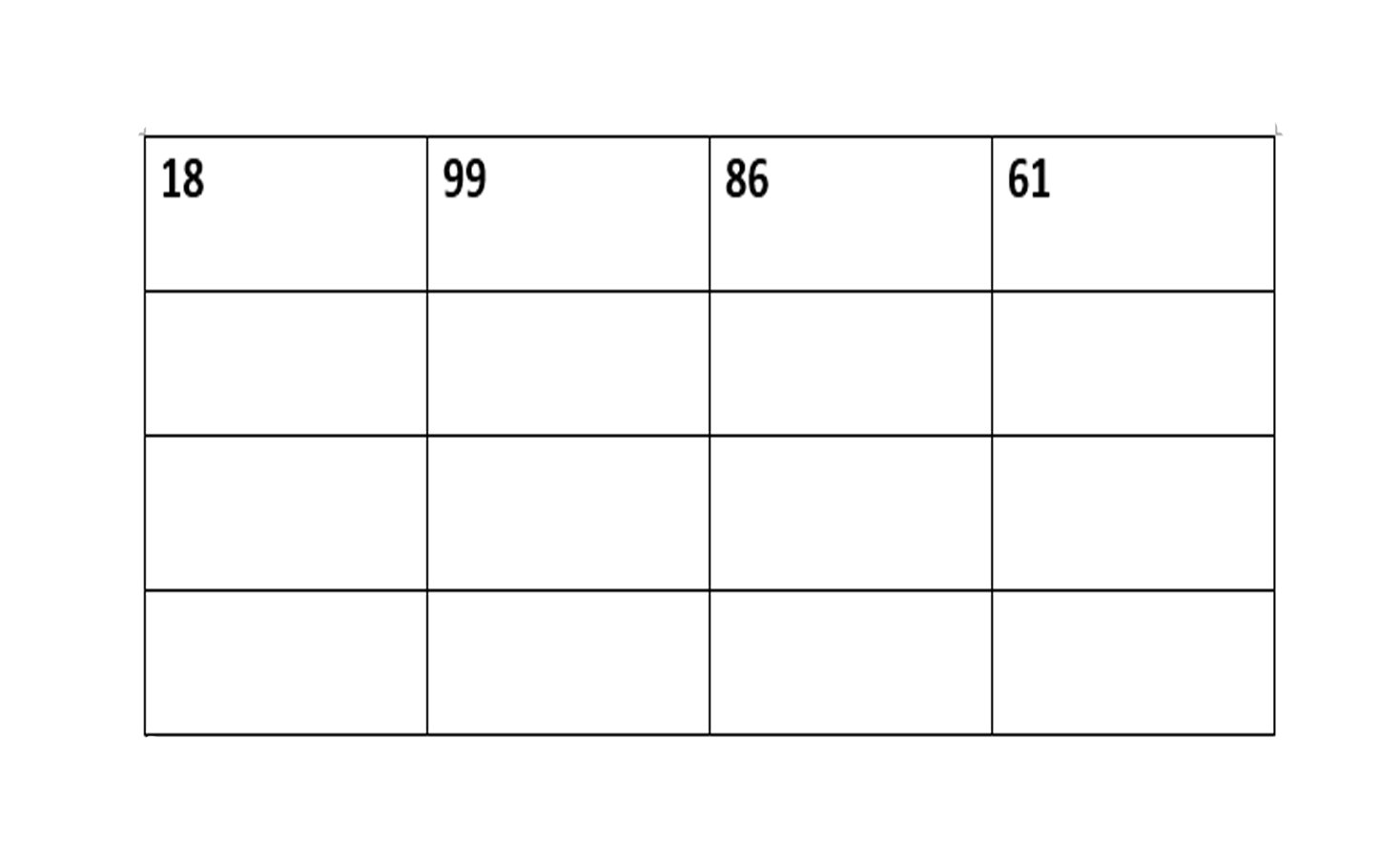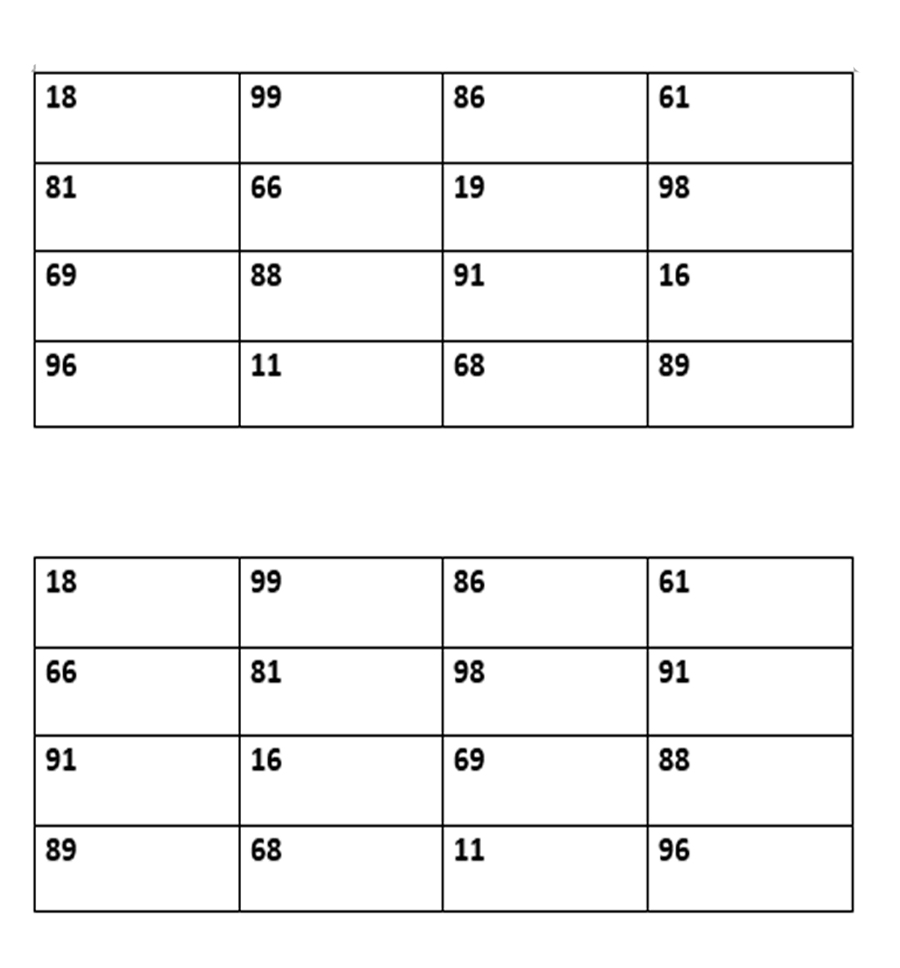# Puzzle 84 | Inverted Matrix

• Difficulty Level : Medium
• Last Updated : 08 Feb, 2018

You have been given the following matrix:Fill in the matrix, in such a way that the sum of the numbers in each row (horizontally, vertically, and diagonally) is 264, even if you hold the matrix upside down. You are only allowed to use the digits 1, 6, 8, and 9, and each number may appear only once in the matrix.

Solution: The possible solutions are:You can see that any two digit number formed by 1,6,8, and 9 , if inverted will also be a valid number. Now, the task becomes easy. Start by trying to form two digit numbers, and different sets by selecting any 4 numbers from them which sums to 264.